Középiskolai Matematikai és Fizikai Lapok
Informatika rovattal
 Már regisztráltál? Új vendég vagy?

# Exercises and problems in Physics November 1997

## New experimental problem:

m. 192. How does the electric power taken up by a pocket radio depend on the volume at which one listens to it?

## New exercises: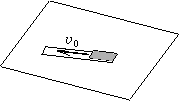FGy. 3099. A long adhesive tape is stuck to a table, then pulled at a uniform speed v0=0.1 m/s backwards by its end turned up. What is the speed of the middle of the moving part of the tape?

FGy. 3100. A 0.5 kg pan mounted on wheels is started at a speed of 0.3 m/s. How does its speed change if 1 kg of sand is `poured' into it on its way?

FGy. 3101. At the end of last year, when the radio announced the unsuccessful launching of the Russian probe Mars '96, it was said that there would be a lot of time for the construction of the next Mars probe. When will the relative positions of Mars and the Earth be the same as at the time of the unsuccessful launching? (A year on Mars is 1.88 times a year on Earth.)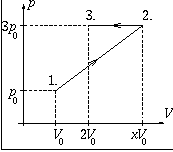FGy. 3102. Some quantity of monatomic ideal gas makes the process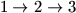shown in the figure. The algebraic sum of the heat taken up and given out by the gas in the course of the process is 7.5p0V0.

a) What is the change in the internal energy of the gas?
b) Determine the value of x.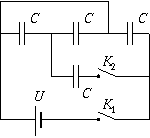FGy. 3103. How does the total energy of the capacitors in the circuit shown in the figure change when first switch K1 and then switch K2, too, is closed?

## New problems:

FF. 3104. Two small bodies, both of charge Q, are fixed at distance l from each other. A small body of charge q and mass m can move along the horizontal straight line connecting the previous two charges. What will the period of the motion of this latter body be when it is displaced a little from its equilibrium position?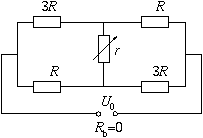FF. 3105. In the circuit shown in the figure R and U0 are given, while r can change, taking up all values.

a) Between what limits does the equivalent resistance change?
b) What is the maximum electric power share of resistor r?

FF. 3106. A 1 m long conical pendulum of 0.5 kg is pulled very slowly upward through a hole drilled into the ceiling. Both at the beginning and at the end of the process the pendulum rotates in a horizontal plane. Initially, the thread is at an angle of 30o with the vertical, while at the end, this angle is 45o. What work has been done during the process?

FF. 3107. A basin is filled in time T1 when the tap at its top is open. It becomes empty in time T2 when the plug-hole at its bottom is open. At what ratio T1/T2 is there a risk of overflow if both the tap and the plug-hole are open?

FF. 3108. The potential of a spherically symmetrical cloud of charges is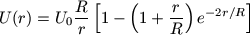. What proportion of the total charge is situated in the part r<R? (Numerical methods can be applied.)